Mechanical Properties of Fluids worksheet

In this page we have Mechanical Properties of Fluids worksheet . Hope you like them and do not forget to like , social share and comment at the end of the page.

Multiple Choice Questions
Question 1: A bowl of soup rests on a table in the dining car of a Rajdhani train. The acceleration of the train is g/4 in the forward direction.
What angle does the surface of the soap make with the horizontal?
a)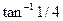b)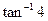c)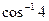d)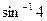Match the column
Question 2:
A block of ice is floating in a liquid of relative density k contained in a beaker
When the ice melts completely, match the column
Column I (k values)
A)  k > 1
B)  k < 1
C)  k=1

Column II
P) Liquid level will increase
Q) Liquid level will decrease
R) Liquid level will remain unchanged
S) No appropriate match given
Question -3
A body of density ρ1 is dropped from rest at a height h into a lake of density ρ221)
Neglect all the dissipative effects
1) Calculate the acceleration of this body while it is in the lake
a)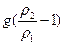Upward direction
b)Downward direction
c)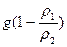Upward direction
d)Downward direction
Question 4 The maximum depth to which the body sinks before returning to float on the surface is proportional to the height h
And it is given by
H=kh.
What is the value of k
a)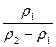b)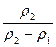c)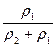d) None of these

Question 5:
Water stands at a depth h behind the vertical face of a dam. It exerts a resultant horizontal force on the dam, tending to slide it along its foundation and a torque tending to overturn the dam about the point X. The width of the dam is L.
The density of the water is ρ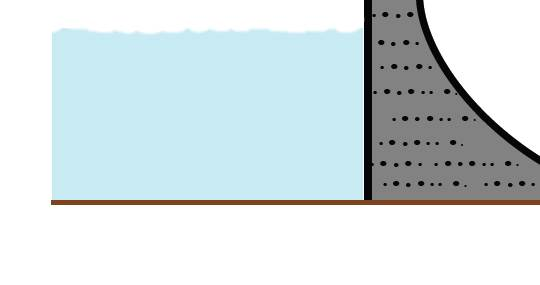1) The resulting horizontal force acting on the dam due to water column
a)  Proportional to h2
b) Proportional to density ρ
c)  Proportional to L
d) None of these
Question 6 The torque of the forces about point X is given by
a)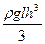b)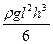c)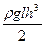d)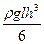Multiple Choice Questions
Question 7
A piston of Weight W has the form of circular disk with radius R1 . The disk has a hole into which a thin walled pipe with a radius R2 is inserted. The piston can enter a cylinder tightly and without friction and it is initially at the bottom of the cylinder. M kg of water is poured into the pipe. Find the height of the piston above the bottom
a)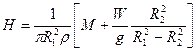b)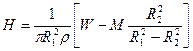c)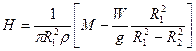d)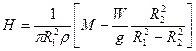Question 8:
A tank contains water on top of mercury. A cube of iron .06 m along each edge is sitting upright in equilibrium in the liquid.
Density of iron =7.7X103 kg/m3
Density of mercury = 13.6X103 kg/m3
Which one of the following is true
a) x=32mm and y=28mm
b) The pressure difference (p2-p1) is 4632 N/m2
c) The weight of the mercury cube is 16.632 g
d) None of these

Download Mechanical Properties of Fluids Worksheet as pdf